# What Does Modern Physics Say About The Number Of Our Dimensions ? - Part 2(Edited)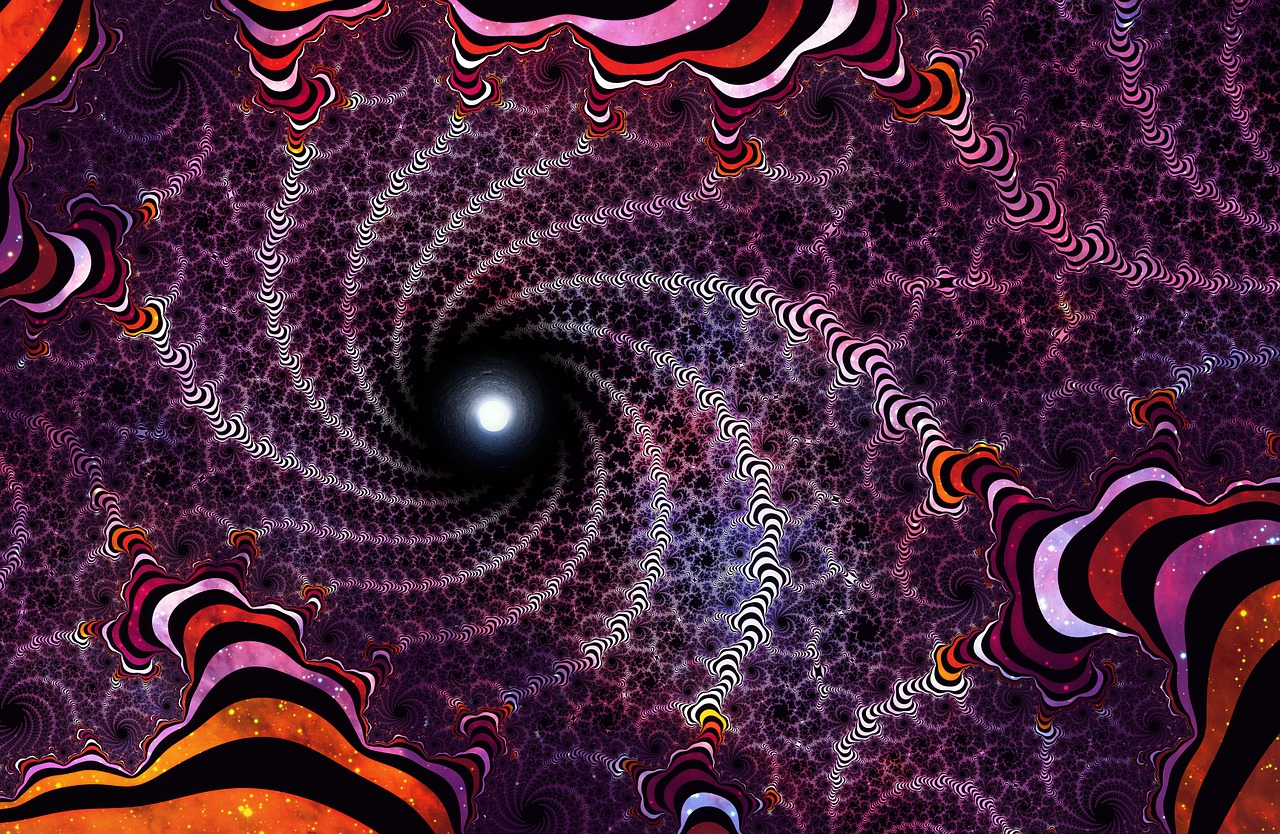Source

In our previous article we discussed what general relativity - an aspect of modern physics, says about the numbers of dimensions in our observable universe, it says that we have four dimensions (3 spatial plus one temporal/time), especially when motion is involved. It was also shown in this same article how gravity arises from the geometric structure of a four-dimensional spacetime, especially in the presence of matter/energy. In this article however, we shall be exploring what another aspect of modern physics (though yet to be standardized because it's predictions are yet to be confirmed experimentally) says about the numbers of dimensions in our universe.

What then is this aspect ?

Well, it basically has to do with the unification of the theory of gravity (general relativity) and that of the other non-gravitational forces (described in modern times by quantum physics) into a single theoretical framework.

Historically, the earliest attempts to unify all the known fundamental forces of nature began around the beginning of the 20th century with notable contributions from Hermann Weyl, Theodor Kaluza and Albert Einstein. As a matter of fact, history has it that during the last days of Einstein's existence on Earth, he become increasingly isolated while trying to get such a theory. Unfortunately, till his eventual death he never succeeded. The theories developed as at that time were almost wholly classical, it unified the theories of the fundamental forces of nature known to physicists as at the time, general relativity for gravity and Maxwell's equations for electromagnetism (both being classical theories). As at that time, quantum physics which would later predict the existence of other forces was still in it's infancy.

Of all the unified theories of that time, that of Kaluza was very distinctive, as it had an extra dimension of spacetime. Mathematically, his theory (Kaluza) was an extension of Einstein field equations, it included an extra space dimension to the four dimensions of spacetime present in the original Einstein field equations, thus making spacetime five-dimensional (four spatial plus one time) and in order to balance the equations on the other side - the part representing the distribution of matter/energy, he added new components that arose from electromagnetism and an unknown scalar field called "dilaton" - a field that was to be discovered experimentally.

With this single theoretical framework, one could get separately the original field equations of general relativity (with four dimensions of spacetime), the original Maxwell's equations and equation(s) corresponding to the new field (dilaton). One consequence of this theory was that we should exist in a five-dimensional universe.

Let's assume this were to be true, that is, in terms of space alone, there are four spatial dimensions in our universe, the question now becomes, why do we observe only three ?

To resolve this problem, a quantum description to Kaluza's theory was given in 1926 by Oskar Klein, this description is referred to today as "compactification". The theory would later be referred to as the "Kaluza-Klein theory".

Unfortunately for the Kaluza-Klein theory, it wasn't a successful theory of everything and it was due to the following reasons

• It was mostly a classical theory

• It could not account for other forces that were later described using quantum physics (strong force, weak force and the quantum electromagnetic force)

• Within it's mathematical framework, there were contradictions with reality

Despite it's failure, it's (amongst other proposed classical unified theories) regarded today as the main precursor to modern candidates for a unified theory of all the fundamental forces - such as string theory, and it's because certain concepts such as "compactification" and "higher than four dimensions of spacetime" were inherited.

The quest for a unified theory of all forces still continued and around the late 20th century, string theory was born, this theory was originally developed to describe the strong force until it was later replaced by quantum chromodynamics, as it (string theory) possessed properties that made it unsuitable for describing the strong force. These properties were later discovered to be useful for describing a quantized version of gravity that was consistent with general relativity.

According to our current understanding of physics, having a theory of quantum gravity that is consistent with general relativity is the first step towards establishing a unified theoretical framework for describing all the known fundamental forces. String theory which can be considered a theory of quantum gravity also possesses properties that makes it a theory of everything, that is, it can describe all other known fundamental forces (strong, gravity, weak and electromagnetic) and for now it seems to be the only way of unifying the theories of all the known forces without much problems - the reason why it's still generally accepted by the physics community.

The first string theory that was developed was called bosonic string theory, this theory could only describe bosons - the family of the three non-gravitational force particles (photons, W and Z bosons and gluons). This theory would later be extended to to describe both bosons and fermions - matter particles, and the resulting theory was called "superstring theory".

What does these string theories really say ?

According to these string theories, particles are not zero-dimensional entities (point-like) but vibrating one-dimensional entities (string-like), they appear point-like at scales lager than the string scale. Just like the different vibrating modes of the strings of a sounding guitar gives rise to different musical tones, the different vibrating modes/states of the string particle gives rise to the properties (especially charge, mass and spin) of the different particles. Superstring theory suggests that a boson particle called "graviton" mediates the gravitational force, so that for the four fundamental forces, we have graviton for gravity, W and Z bosons for weak force, photon for electromagnetic force and gluons for strong force.

For these theories to be successful mathematically, one requirement is that we should exist in a universe with higher than four dimensions of spacetime. For bosonic string theory, we should exist in 26 dimensions of spacetime, that is, our usual four-dimensional spacetime (3 spatial plus one time dimension) and extra 22 spatial dimensions (sometimes called a hyperspace), making it total of 26 dimensions (22 + 4)

Hmmm.... I'm still wondering how an object with 26 dimensions would look like, it's going to be mind-blowing.

In the case of superstring theory, we should exist in a ten-dimensional spacetime, that is, our usual 4D spacetime plus 6 extra spatial dimensions (also a hyperspace). We should note that superstring theory has different versions and they all can be traced to a generalized theoretical framework called M-theory. According to M-theory, we should exist in a eleven-dimensional spacetime (4D spacetime + 7 spatial dimensions).

To explain why we only observe 4D spacetime (with time) or 3D space (without time), superstring theory presents us with two options, namely compactification and brane-world scenario.

In the case of compactification, the extra 6 dimensions of space in the total 10 dimensions of spacetime are shaped (especially in a curled up manner) in such a way that at large/distant scales the 10 dimensions of spacetime appears as 4 dimensions of spacetime - the six dimensions of space appears not to exist, and that the shape taken by these 6 extra dimensions of space depends on the physical scenario.
A simple example is the case of the pipe in the image below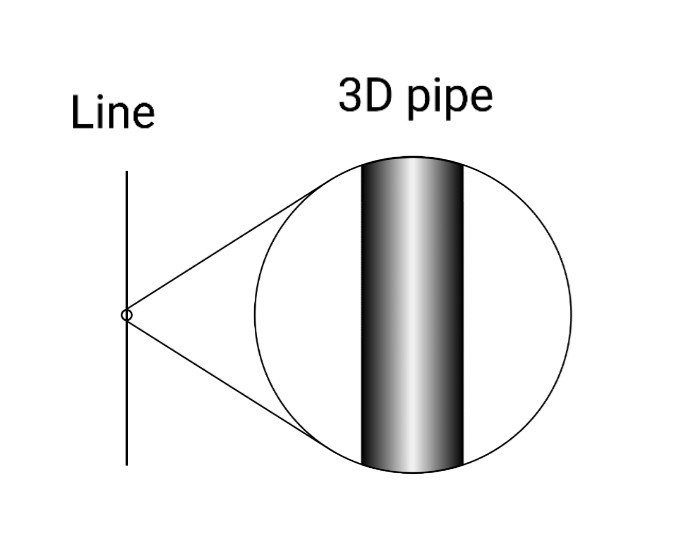Source

The pipe is actually three-dimensional, one dimension represents the length of the pipe (it is straight, from up to down of the above image), the other two dimensions which are supposed to represent it's width and depth now makes up the circular part of the pipe. If you happened to be far away from the pipe, the pipe would appear one-dimensional (like a line) - see the left part of the image above, it's as if the other two dimensions no longer exist. When you move closer to/magnify the line, the other dimensions becomes visible.

The act of the other two dimensions (representing the width and depth of the pipe) taking shapes (circular) such that at distant scales they appear not to exist is referred to as "compactification".

In physics, for superstring theory to predict all that the standard model of particle physics predicts in 4D spacetime, the compactified shape of the extra 6 spatial dimensions (remember superstring theory requires us to exist in 10-dimensional spacetime) should be that given in the image below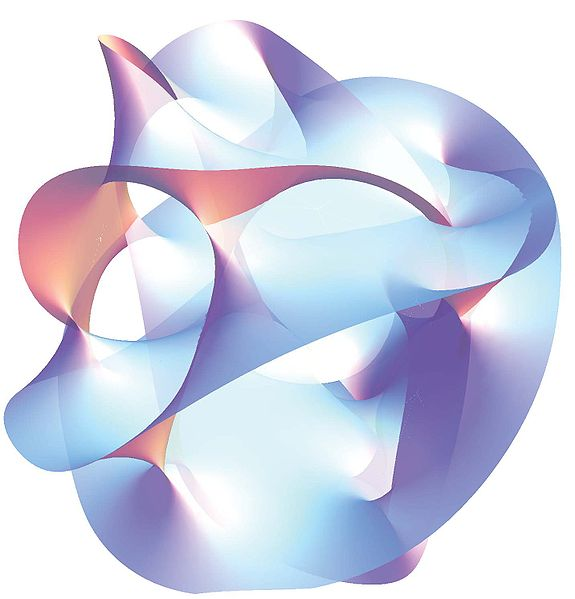Source

The shape above is known as the Calabi-Yau manifold.

In the case of brane-world scenario, we exist in a four-dimensional spacetime that is itself a subspace of higher-dimensional spacetime (10 in the case of superstring theory). To understand this, let's observe the tesseract - a four-dimensional cube, below or in our previous articleSource

This tesseract can be visualized as being made up of 8 cubes, as seen below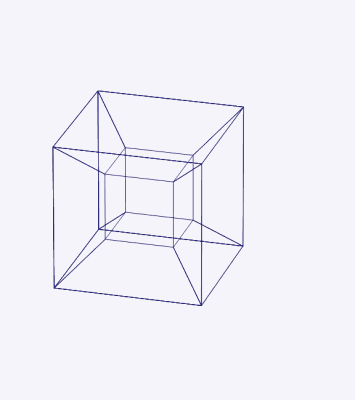Source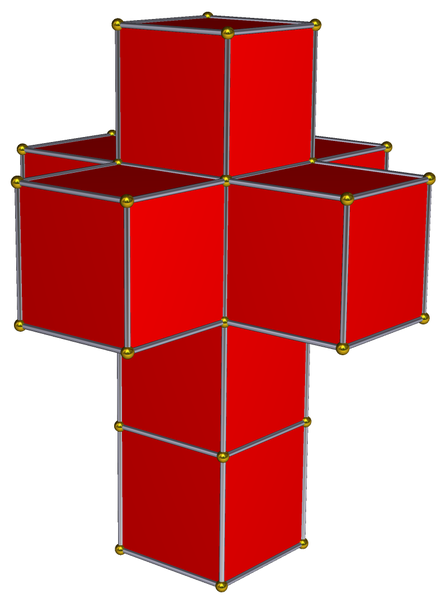Source

If we exist "inside" one of the 8 cubes, then we exist in a 3D spacetime (a cube) that is itself a subspace of the tesseract (4D spacetime). If we exist "on the surface" of one of the 8 cubes, then we exist in a 2D spacetime (a square) that is itself a subspace of the tesseract (4D spacetime).

This brane-world scenario also explains why gravity is the weakest of all the four fundamental forces by suggesting that gravity propagates in all of the ten dimensions of spacetime whereas the other three propagates in our familiar 4D spacetime. The idea there is that the gravitational field has become diluted with the presence of the extra 6 dimensions of space, just like a sugary drink can get diluted when added extra water.

As interesting as string theory and it's variants are, it's predictions such as the extra dimensions of space, multiverse, supersymmetry and others are yet to be confirmed experimentally, thus preventing it from being a viable candidate for a unified theory of everything. However, one conclusion that can be drawn from this article is that, if there's going to be another theory that attempts to unify the theory of gravity with that of the other forces, then there's a possibility that it may still suggest that we exist in a universe with higher than four dimensions of spacetime.

Maybe it's true that we indeed exist in such a universe (with more than four dimensions of spacetime) and that we maybe be looking at things from the wrong perspective - theoretically I mean, maybe it's not true at all, it could probably be that we are over mathematizing things but one fact remains and it's that time would eventually tell.

It's here we conclude this article, have a thoughtful day and see you next time.

Kaluza–Klein theory

String theory

How the search for a unified theory stumped Einstein to his dying day

Albert Einstein

Thank you all once again for stopping by to read my jargons and also thank you @juecoree, @lemouth and the @Steemstem team for your valuable supports.Source

Lastly, please don't forget to do the needful
Upvote
Comment
Reblog
If you enjoyed my jargons.0
0
0.000This is a very interesting blog I smiled a bit when I saw the image of the tube to explain higher dimensions, as I use the same kind of pictures with students when I need to explain how extra dimensions look like. Instead of a tube, I however use a pencil.

Anyways, as you said it, unification is a researched goal for a long time. Already at the level of the three fundamental interactions of the Standard Model (i.e. without gravity), there is already a lot to understand and test. As soon as we combine these three forces, we have indeed a lot of new particles and phenomena around the place that are in principle observable (and thus testable). The flavour anomalies and their possible leptoquark interpretation are in this respect at least intriguing.

The only conclusion is however yours: the future (and data) will tell us.

0
0
0.000Thank you for including further reading as this is beyond fascinating. I always knew there were more than four dimensions but I never imagined 10.

0
0
0.000Thanks for your contribution to the STEMsocial community. Feel free to join us on discord to get to know the rest of us!

Please consider delegating to the @stemsocial account (85% of the curation rewards are returned).

You may also include @stemsocial as a beneficiary of the rewards of this post to get a stronger support.

0
0
0.000